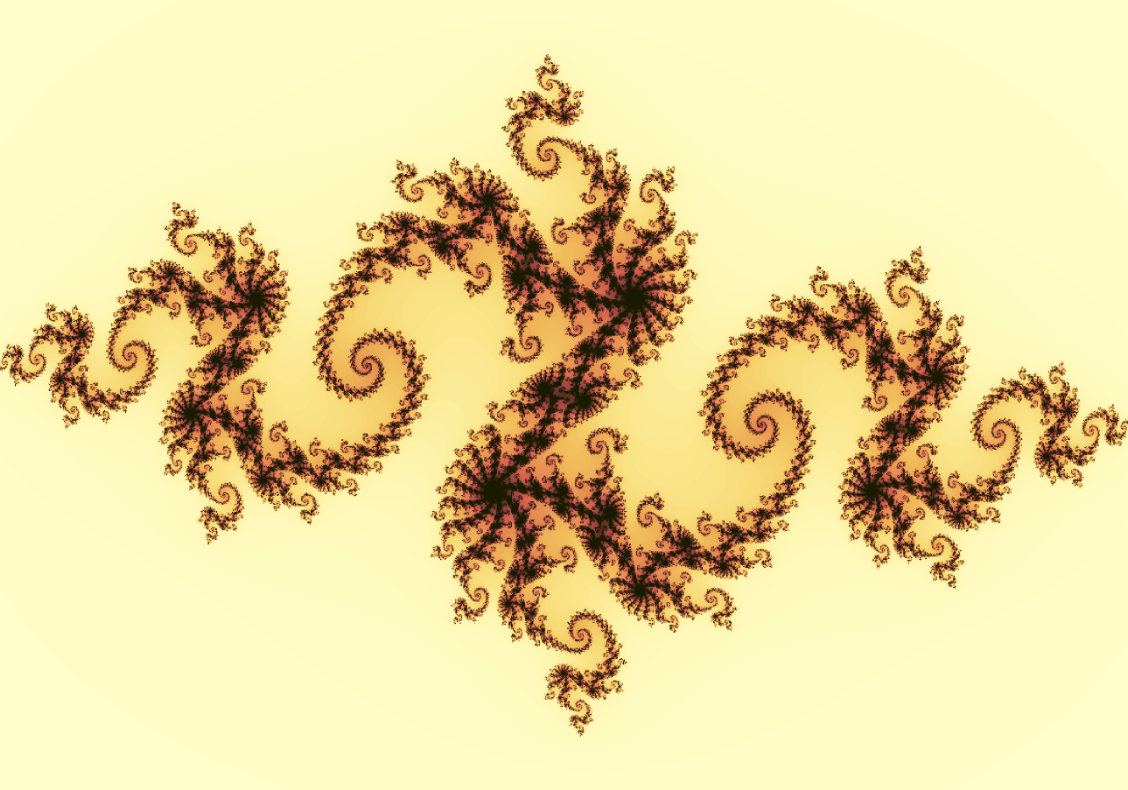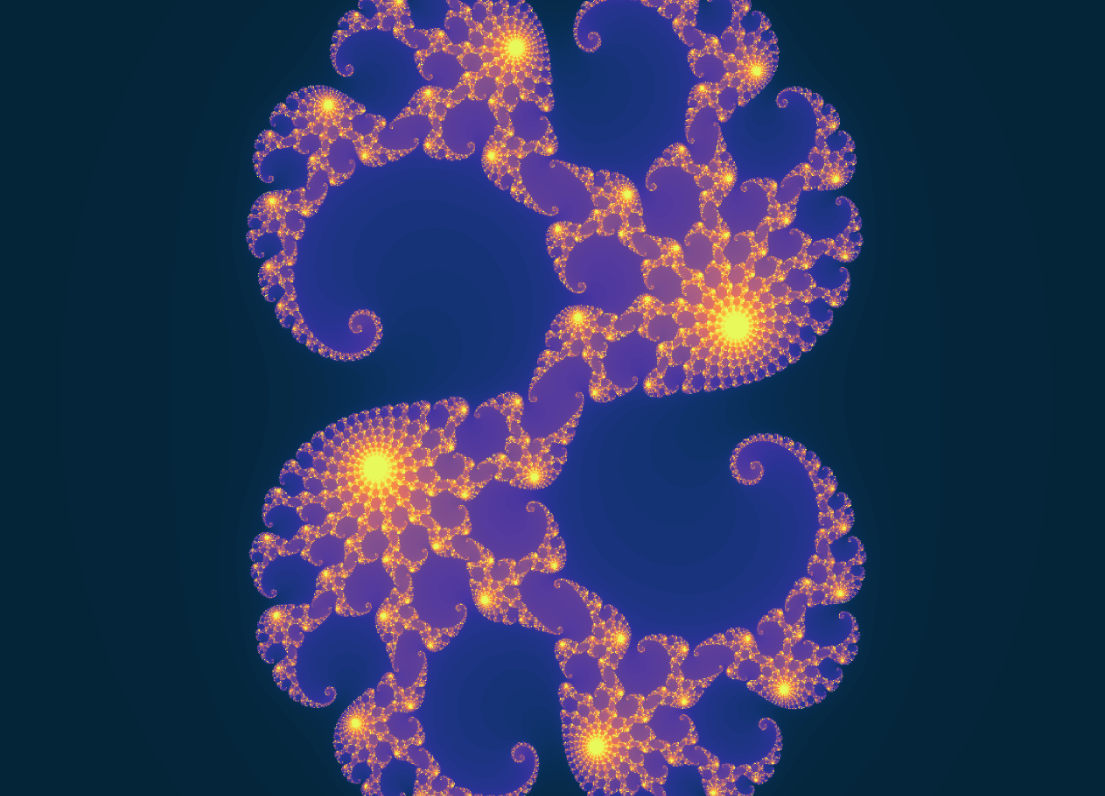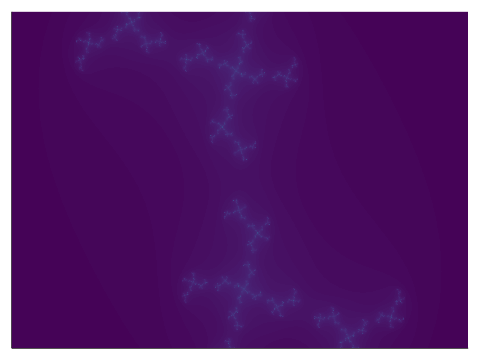# Fun with Complex Quadratic Polynomial Julia Set by Using Julia Programing

A Julia set is an area boundary formed by points in the complex number plane or the Riemann sphere
Julia Programming
Mathematics
Data Visuliazation
Data Science
Author

Steven Wang

Published

July 29, 2022

## What is a Julia Set

Julia set was named after the French mathematician Gaston Julia, who studied the set in the early 20th century. Generally speaking, a Julia set is an area boundary formed by points in the complex number plane or the Riemann sphere (named after Bernhard Riemann, is a model of the extended complex plane: the complex plane plus one point at infinity) that diverge to infinity and those that remain finite under repeated iteration of some mapping (function). One particular Julia set with quadratic polynomials expression as in Equation 1 was risen to a well-known Mandelbrot set, where all points of the Julia set are connected.

The Julia set consists of values where a very small change in the c value can cause dramatic value changes in the sequence of iterated function outcomes. Therefore, the behavior of Julia sets is very turbulent, subject to the parameter c value change.

Julia set fractals are usually generated by initializing a complex number:

z = x + {y}\times{i}

where i is imaginary unit such that

i^2 = -1

and x and y are real numbers represents image pixel coordinates in the range of about -2 to 2. Then, z is repeatedly updated using:
f(z) = z^2 + c \tag{1} where c is another complex number that gives a specific Julia set. After numerous iterations, if the magnitude of z is less than 2 we say that pixel is in the Julia set and color it accordingly. Performing this calculation for a whole grid of pixels gives a fractal image.

## Complex Quadratic Polynomial Julia Sets Examples

Code
function juliaset(z, c, R, N)
n = 0
while n <= N && abs(z) <= R^2 - R
n += 1
z = z^2 + c
end
return n > N ? 0 : n/N
end

R, N, L, K = (2, 1000, 1500, 1000)
x = range(-1.5, 1.5; length = L)
y = range(-1.0, 1.0; length = K)
A = zeros(K, L)

### Example 1: c = -0.79 + 0.15i

Julia set for f(z) = z^2 + c, where c = -0.79 + 0.15i

See Figure 1.

Code
c = -0.79 + 0.15im
A = @. juliaset(x' + y * im, c, R, N)

using Plots #, PyPlot
# pyplot()
heatmap(A;
c = :lajolla,
clims = (0, 0.15),
cbar = :none,
axis = :none,
ticks = :none,
)Figure 1: Julia Set for c = -0.79 + 0.15i, color scheme = lajolla

### Example 2: c = 0.28 + 0.008i

Julia set for f(z) = z^2 + c, where c = 0.28 + 0.008i

See Figure 2.

Code
c = 0.28 + 0.008im
A = @. juliaset(x' + y * im, c, R, N)

using Plots #, PyPlot
# pyplot()
heatmap(A;
c = :thermal,
clims = (0, 0.15),
cbar = :none,
axis = :none,
ticks = :none,
)Figure 2: Julia Set for c = 0.28 + 0.008i, color scheme = thermal

### Example 3: c = -0.4 + 0.6i

Julia set for f(z) = z^2 + c, where c = -0.4 + 0.6i

See Figure 3.

Code

c = −0.4 + 0.6im
A = @. juliaset(x' + y * im, c, R, N)

using Plots #, PyPlot
# pyplot()
heatmap(A;
c = :haline,
clims = (0, 0.15),
cbar = :none,
axis = :none,
ticks = :none,
)Figure 3: Julia Set for c = −0.4 + 0.6i, color scheme = haline

## Julia Sets Animation

We will now create an animation of the Julia sets for c defined as follows

C_\alpha = 0.7885 \exp^{ \alpha \cdot i }, \qquad \alpha \in \left [\frac{\pi}{2}, \frac{3\pi}{2} \right ].

See the GIF animation as in Figure 4.

Code
C = @. 0.7885 * exp(range(π/2, 3π/2; length = 500) * im)

anim = @animate for c in C
A = @. juliaset(x' + y * im, c, R, N)
heatmap(A;
c = :viridis,
clims = (0, 0.15),
cbar = :none,
axis = :none,
ticks = :none,
size = (600, 480),
)
end
gif(anim, "jset_quadratic.gif", fps = 20)Figure 4: Julia Set Animation, color scheme = viridis

## References

“Gaston Maurice Julia | French Mathematician | Britannica.” n.d. Accessed August 4, 2022. https://www.britannica.com/biography/Gaston-Maurice-Julia.
“Julia Set.” 2022. In Wikipedia. https://en.wikipedia.org/w/index.php?title=Julia_set&oldid=1068868935.
“Julia Set – from Wolfram MathWorld.” n.d. Accessed August 4, 2022. https://mathworld.wolfram.com/JuliaSet.html.
“Julia Set | Mathematics | Britannica.” n.d. Accessed August 4, 2022. https://www.britannica.com/science/Julia-set.
“Mandelbrot Set.” 2022. In Wikipedia. https://en.wikipedia.org/w/index.php?title=Mandelbrot_set&oldid=1101257069.
“Understanding Julia and Mandelbrot Sets.” n.d. Accessed August 4, 2022. http://www.karlsims.com/julia.html.